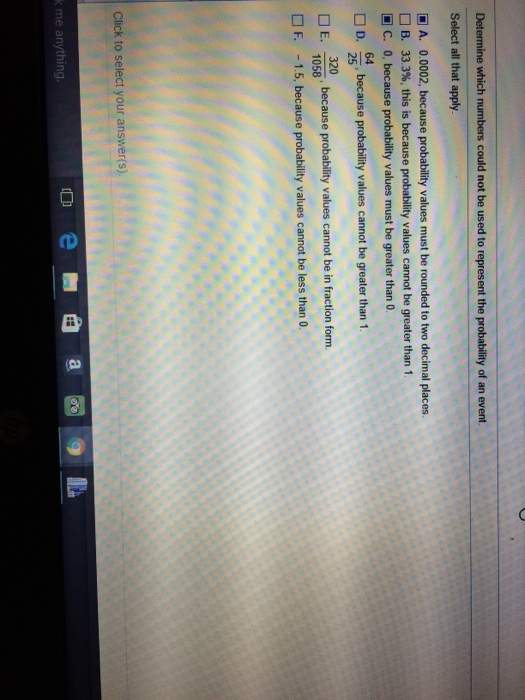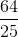# Determine which numbers could not be used to represent the probability of an event.Determine which numbers could not be used to represent the probability of an event. Select all that apply A. 0.0002, because probability values must be rounded to two decimal places. B. 33.3%, this is because probability values cannot be greater than 1 C. 0, because probability values must be greater than 0 D. 64/25, because probability values cannot be greater than 1 E. 320/1058, because probability values cannot be in fraction form. F. -1.5, because probability values cannot be less than 0.

P: is probability
It can take any value [0,1] (0,1 are included)
And also it can take any value [0% ,100%] ( 0% and 100%
included)
It can not take negative Values.
The numbers which can not be used to represent the probability
of an evens are
D.
because probability Values cannot be greater than 1.
F. -1.5, because probability Values cannot be less than
0.